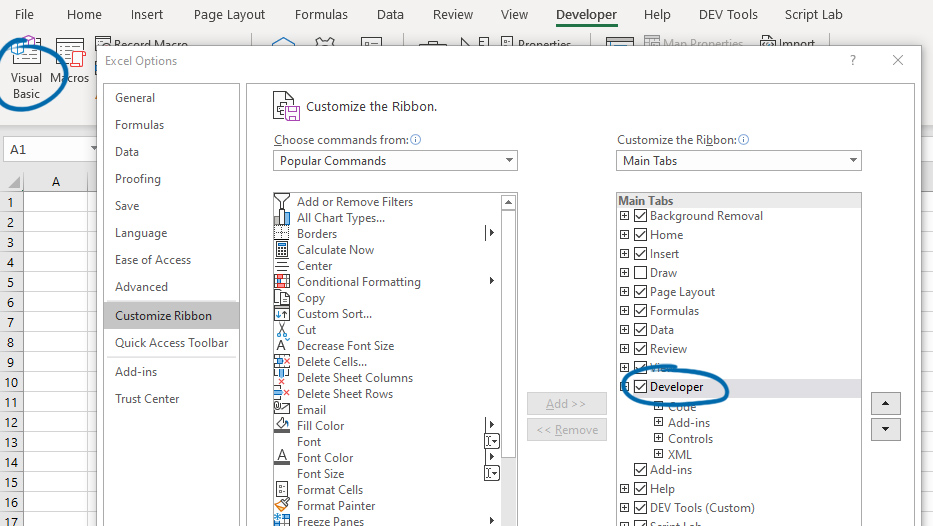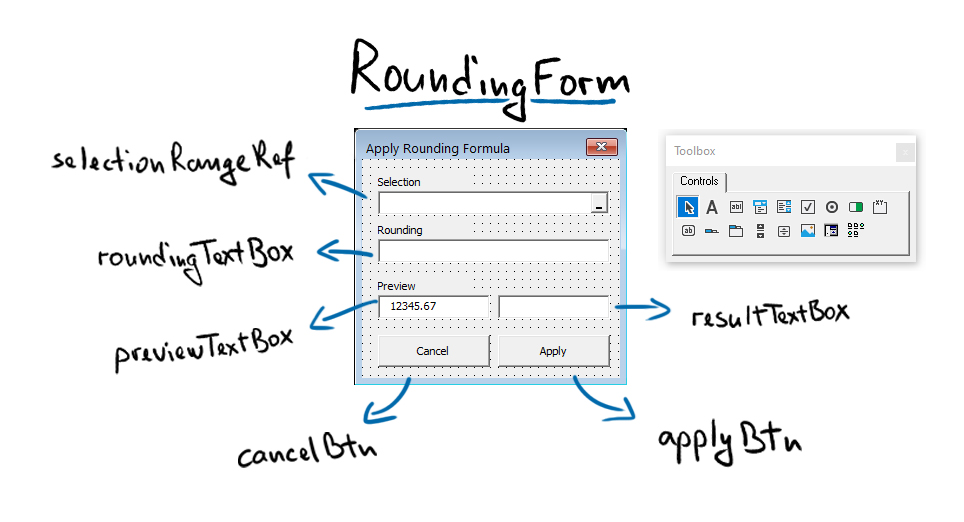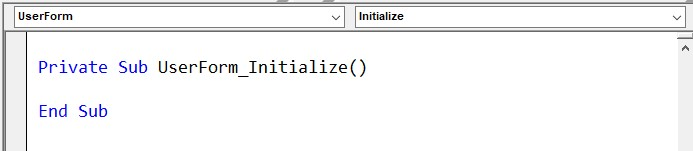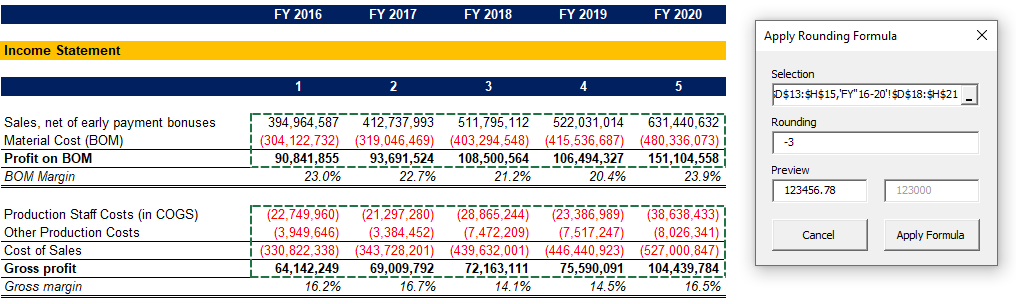## Add Rounding to Multiple Cells in Excel – VBA Tool Dev

Lately, I’ve been working on a side project, modeling some financial statements. When I do those, I like to work with full numbers, as I get a better feel of the material items. However, when it’s time to prepare the final deliverables, I always round in thousands.

What I have been doing so far is going in and wrapping the formulas by hand. I like using Excel’s round formula with -3 decimal places. This means that it rounds it to thousands but keeps the zeros, so you still get a good feel of the figures’ magnitude.

For example, ROUND(123456,-3) will give us 123000.

I’ve had enough of that manual adding of the ROUND formula! So, I decided to write a simple tool to help me automate the task. I’ve been using it for two weeks now, and it has saved me quite some time, so I decided to share how you can do it. It’s quite simple, and the best part is, you can modify it to wrap cells with any formula you want!

### Enable the Developer tab

Before we start, we need to enable the Developer tab to access the Visual Basic Editor. To do so, go to Options -> Customize Ribbon, and tick the Developer tab. You will then see the Visual Basic editor icon.## Designing our User Form

Before we start writing the code, we need to visualize our user form.

Open the VB editor and add a new UserForm to your PERSONAL.XLSB file. This is the file that’s always open in the background whenever you run Excel. If you store Macros and UserForms here, they will always be available when you work on different files.

Suppose you don’t see your PERSONAL.XLSB file, you have to go back to Excel and record at least one Macro (make sure to choose Store Macro in Personal Macro workbook). It doesn’t matter what it does, you can delete it later, but the first time you record a Macro, it will create your PERSONAL.XLSB file.

Once we create a new UserForm, we have to design it. For this project, I settled on something like this:Apart from setting the objects’ names, I also marked Cancel = True for the Cancel button and made sure the resultTextBox is disabled and locked.

For the selectionRangeRef object, we use a RefEdit object. This is not visible by default in some versions of Excel. You can right-click somewhere on the Toolbox and click Additional Controls, where you can find it and mark it.## Get a FREE Excel Benchmark Analysis Template

For your reference, here are screenshots of the specific settings for each field:

## Writing the Code

Once we have designed our UserForm, we can start adding some code. I always start with the Cancel button, so I can quickly exit the form when testing.

### Cancel Button

``````Private Sub cancelBtn_Click()
End Sub``````

Double click on the Cancel button, and it will open up the code editor and add a Click() event to our cancelBtn object. The code in this sub-routine (where Sub comes from) is pretty simple – it just unloads the current UserForm.

### Form Initialize

``````Private Sub UserForm_Initialize()
roundingTextBox.Value = GetSetting("MagnimetricsTools", "ApplyRounding", "roundingFormat", 0)
End Sub``````

We will be saving our rounding format field to the registry to be available whenever we rerun the Macro. That way, it will be easier to keep the work consistent across our models.

Therefore, we will use the initialization event for the UserForm to load the setting. It’s okay, and we can type the code to save it later on. This is what the GetSetting() method does. It takes a few arguments – the app name, section name, key name, and a default value, in our case – zero.

You can add various event handlers by using the object and event dropdowns at the top of your code editor.### Check for Valid Range

``````Function isValidRange(rngString As String) As Boolean
Dim rng As Range
On Error Resume Next
Set rng = ActiveWorkbook.ActiveSheet.Range(rngString)
If Err.Number <> 0 Then
Exit Function
End If
isValidRange = True
End Function``````

We define a function similar to the way we define a sub-routine. The major difference is that a function returns a value.

Our isValidRange will return a Boolean value – True if the string we provide is an acceptable range and False if it’s not.

We use the ‘On Error Resume Next’ expression, which ensures if the code runs into an error, it will continue executing the next line and not throw up an exception to the user.

We try to create a valid range out of the provided string. We then see if this produced errors. If it did (Err.Number is different from 0), we Exit the function, meaning isValidRange returns False. If there are no errors, the function continues and sets itself to True (we have provided a valid range).

### Apply Button

``````Private Sub applyBtn_Click()
Dim selectionRange As Range
Dim cell As Range
Dim oldFormula As String
Dim newFormula As String
Dim roundingFormat As Variant

SaveSetting "MagnimetricsTools", "ApplyRounding", "roundingFormat", roundingTextBox.Value

roundingFormat = roundingTextBox.Value
If IsNumeric(roundingFormat) Then
If roundingFormat <> Int(roundingFormat) Then
roundingFormat = Round(roundingFormat, 0)
End If
Else
roundingFormat = 0
roundingTextBox.Value = 0
End If

If Not isValidRange(selectionRangeRef.Value) Then
selectionRangeRef.Value = ""
selectionRangeRef.SetFocus
MsgBox "Error", vbCritical, "Selection Error"
Exit Sub
End If

Set selectionRange = ActiveSheet.Range(selectionRangeRef.Value)

For Each cell In selectionRange

If cell.HasFormula Then

oldFormula = cell.Formula

'check if formula already has rounding
If Left(cell.Formula, 6) <> "=ROUND" Then
'wrap formula with round
newFormula = "=ROUND(" & Right(oldFormula, Len(oldFormula) - 1) & "," & roundingFormat & ")"
'MsgBox newFormula
cell.Formula = newFormula

Else
End If

ElseIf IsNumeric(cell) Then
'wrap number with round
newFormula = "=ROUND(" & cell.Value & "," & roundingFormat & ")"
'MsgBox newFormula
cell.Formula = newFormula
End If

Next cell

End Sub``````

Let’s break down the above sub-routine code into chunks and go over what it does. We start by defining some variables.

``````    Dim selectionRange As Range
Dim cell As Range
Dim oldFormula As String
Dim newFormula As String
Dim roundingFormat As Variant``````

We define roundingFormat as a Variant, meaning it can hold any value. We do so because the user might try to input text in the field, and we don’t want this to cause the Macro to break.

We then proceed to save our rounding format to the registry.

``    SaveSetting "MagnimetricsTools", "ApplyRounding", "roundingFormat", roundingTextBox.Value``

The next section of the code will check if the rounding format we have provided is an integer, which is what the rounding function will need for the number of digits after the decimal point. We will check if the value of the roundingTextBox input field is numeric. If it is, we will check if it’s an integer (a full number) and round it if it’s not. On the other hand, if the value is not numeric, we will set it to zero (and update the input field).

``````    roundingFormat = roundingTextBox.Value
If IsNumeric(roundingFormat) Then
If roundingFormat <> Int(roundingFormat) Then
roundingFormat = Round(roundingFormat, 0)
End If
Else
roundingFormat = 0
roundingTextBox.Value = 0
End If``````

After ensuring our rounding format is proper, we will utilize the isValidRange function to ensure the user has provided an acceptable range.

We will see if the range is Not valid, and if that’s the case, we will erase the RefEdit field, set the user focus on it, and show an error message. We will also Exit the Sub, which will terminate the sub-routine before it executes the following code.

``````    If Not isValidRange(selectionRangeRef.Value) Then
selectionRangeRef.Value = ""
selectionRangeRef.SetFocus
MsgBox "Error", vbCritical, "Selection Error"
Exit Sub
End If``````

Once we’ve taken care of this, we will set our selection range.

``    Set selectionRange = ActiveSheet.Range(selectionRangeRef.Value)``

Now that we have the range we want to process, it’s time to loop through each cell in it. We do this with a For-Each loop.

``````    For Each cell In selectionRange

If cell.HasFormula Then

oldFormula = cell.Formula

'check if formula already has rounding
If Left(cell.Formula, 6) <> "=ROUND" Then
'wrap formula with round
newFormula = "=ROUND(" & Right(oldFormula, Len(oldFormula) - 1) & "," & roundingFormat & ")"
'MsgBox newFormula
cell.Formula = newFormula

Else
End If

ElseIf IsNumeric(cell) Then
'wrap number with round
newFormula = "=ROUND(" & cell.Value & "," & roundingFormat & ")"
'MsgBox newFormula
cell.Formula = newFormula
End If

Next cell``````

For each cell, we check if it has a formula or is numeric. Otherwise, we will do nothing (in case it’s empty or has text in it.

If it has a formula, we will first see if it starts with “=ROUND”. If it does, the cell was already rounded, and we will do nothing. But if it’s different, we will use the oldFormula string to reconstruct it wrapped in a ROUND function by trimming the equal sign from the original formula and using the roundingFormat variable we set previously.

On the other hand, if the cell is numeric, we will directly wrap the cell value in a ROUND formula.

After the sub-routine has processed all cells in our selection, we can go ahead and unload the UserForm.

``    Unload Me``

### Building our Preview

``````Private Sub previewTextBox_Change()

Dim roundingFormat As Double
Dim previewNum As Double

roundingFormat = roundingTextBox.Value
If IsNumeric(roundingFormat) Then
If roundingFormat <> Int(roundingFormat) Then
roundingFormat = Round(roundingFormat, 0)
End If
Else
roundingFormat = 0
End If

previewNum = CDbl(previewTextBox.Value)

previewNum = WorksheetFunction.Round(previewNum, roundingFormat)

resultTextBox.Value = previewNum

End Sub``````

Let’s add some code to take care of our preview. We will add a default value directly in the field and then add a Change() event to the previewTextBox object.

We start by defining two variables for our rounding format and the preview number.

We will make sure we have a numeric value for the rounding format, as we already did before. We then convert the preview number (the one we can edit) to a double and round it. We use the WorksheetFunction, as the VBA round function doesn’t work negative decimal places parameter.

### Fixing the Preview

``````Private Sub roundingTextBox_Change()
If IsNumeric(roundingTextBox.Value) Then
previewTextBox_Change
End If
End Sub``````

However, we want the preview to change whenever we change our rounding format as well. Therefore, we add a Change() event to the decimal places’ input field.

To make sure it only runs when the rounding format is an actual value, we add a check if it’s a numeric value. That way, when we start typing negative three (-3) to round to thousands, the code won’t throw an error when we input the negative sign. However, as soon as we add the three, it’s now a negative number, and the code will run.

## Trying It Out

Let’s see how our newly developed tool works in real life!

Here we have a model that we want to present rounded to thousands, but we want the numbers to remain intact. We run our Macro and make our selection:## Conclusion

Once we have our selection, we type -3 as our Rounding parameter and hit the Apply Formula button. Voila!Being able to automate simple repetitive tasks in Excel is fantastic! And VBA makes it relatively easy to achieve. You can start with what we have achieved so far and extend and tailor it to your needs.

Please, show your support by sharing the article with colleagues and friends.

You can take download the full VB code here to get a better understanding of the flow:## FCCA, FMVA

Hi! I am a finance professional with 10+ years of experience in audit, controlling, reporting, financial analysis and modeling. I am excited to delve deep into specifics of various industries, where I can identify the best solutions for clients I work with.

In my spare time, I am into skiing, hiking and running. I am also active on Instagram and YouTube, where I try different ways to express my creative side.

The information and views set out in this publication are those of the author(s) and do not necessarily reflect the official opinion of Magnimetrics. Neither Magnimetrics nor any person acting on their behalf may be held responsible for the use which may be made of the information contained herein. The information in this article is for educational purposes only and should not be treated as professional advice. Magnimetrics and the author of this publication accept no responsibility for any damages or losses sustained in the result of using the information presented in the publication.